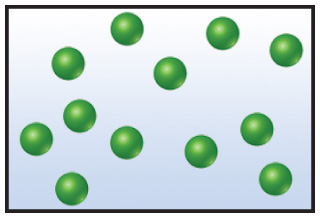# Problem: Consider the sample of gas depicted below:What would the drawing look like if the volume and temperature remained constant while you removed enough of the gas to decrease the pressure by a factor of 2?

###### FREE Expert Solution

We have to find out what would this picture look like if we wanted to decrease the pressure by a factor of 2.

This means that we have to establish a relation between pressure and amount of a gas.

Recall from the ideal gas equation:It could be rearranged as:###### Problem Details

Consider the sample of gas depicted below:What would the drawing look like if the volume and temperature remained constant while you removed enough of the gas to decrease the pressure by a factor of 2?